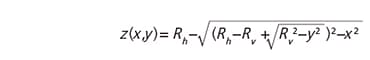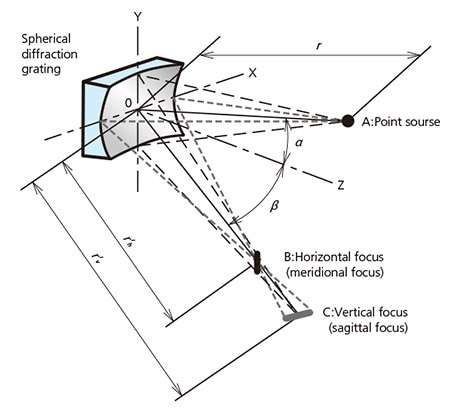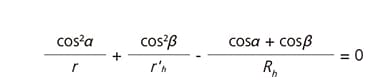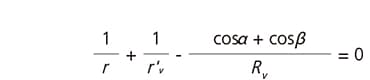Toroidal Diffraction Gratings

A toroidal diffraction grating is a sort of concave gratings. The toroidal shape is defined by two curvature radii at each central axis. Toroidal shapes where Rh is a horizontal curvature radius and Rv is a vertical curvature radius. The toroidal shapes are expressed in the following equation.The paraxial focal positions of a toroidal grating in Fig.8 are defined by equations (17) and (18). Here r is the entrance length, r'h is the horizontal focal length, and r'v is the vertical focal length. α and β satisfy the grating equation (2)'.Fig.8 The different focus of the Spherical diffraction gratingThe spherical diffraction gratings are formed on spherical blanks( Rh=Rv ) that has two focal lengths(r'hr'v ) has astigmatisms, The toroidal diffraction gratings are formed on toroidal (aspheric) blanks( RhRv ) that can correct astigmatisms.